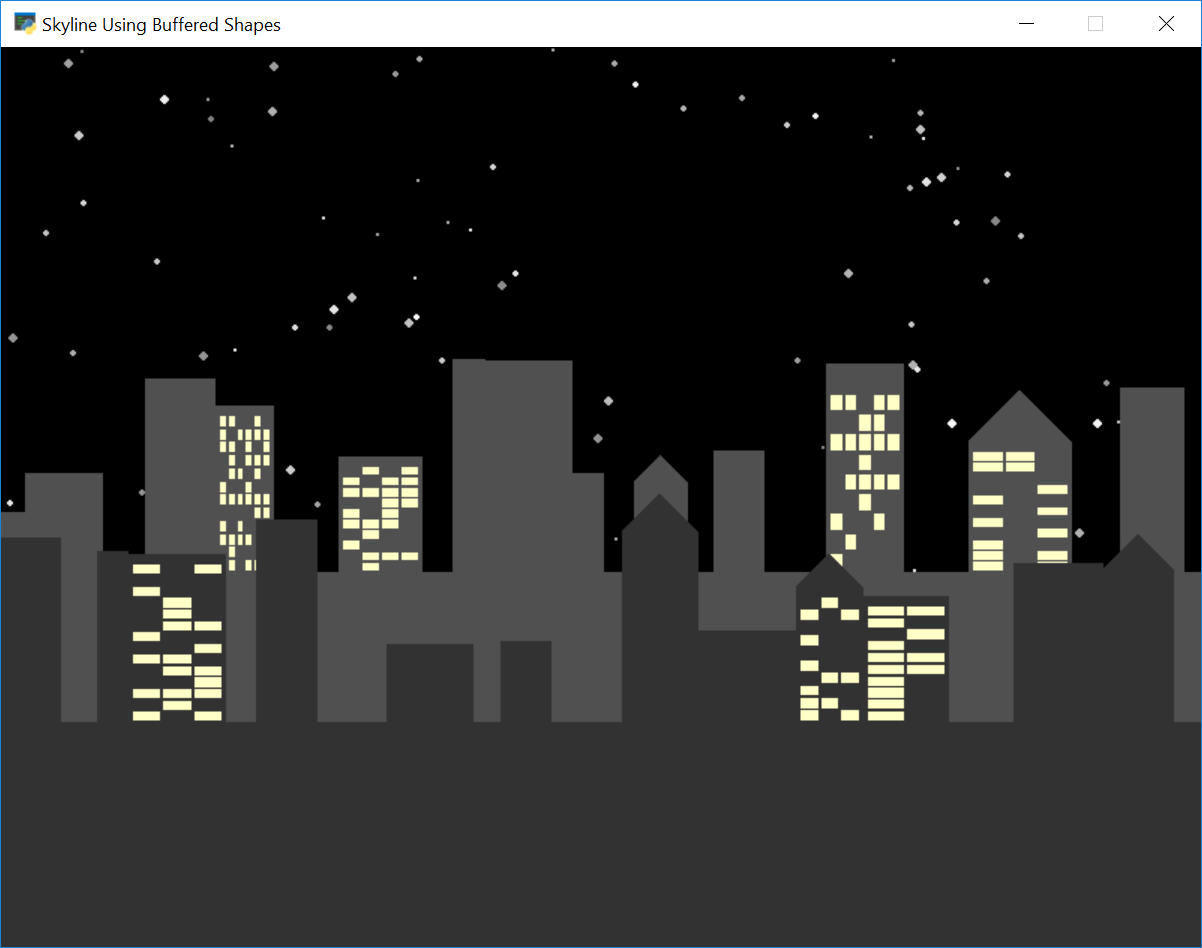# Shape List - Skylines#shape_list_demo_skylines.py#
 ``` 1 2 3 4 5 6 7 8 9 10 11 12 13 14 15 16 17 18 19 20 21 22 23 24 25 26 27 28 29 30 31 32 33 34 35 36 37 38 39 40 41 42 43 44 45 46 47 48 49 50 51 52 53 54 55 56 57 58 59 60 61 62 63 64 65 66 67 68 69 70 71 72 73 74 75 76 77 78 79 80 81 82 83 84 85 86 87 88 89 90 91 92 93 94 95 96 97 98 99 100 101 102 103 104 105 106 107 108 109 110 111 112 113 114 115 116 117 118 119 120 121 122 123 124 125 126 127 128 129 130 131 132 133 134 135 136 137 138 139 140 141 142 143 144 145 146 147 148 149 150 151 152 153 154 155 156 157 158 159 160 161 162 163 164 165 166 167 168 169 170 171 172 173 174 175 176 177 178``` ```""" City Scape Generator If Python and Arcade are installed, this example can be run from the command line with: python -m arcade.examples.shape_list_skylines """ import random import arcade import time SCREEN_WIDTH = 1200 SCREEN_HEIGHT = 600 SCREEN_TITLE = "Skyline Using Buffered Shapes" def make_star_field(star_count): """ Make a bunch of circles for stars. """ shape_list = arcade.ShapeElementList() for star_no in range(star_count): x = random.randrange(SCREEN_WIDTH) y = random.randrange(SCREEN_HEIGHT) radius = random.randrange(1, 4) brightness = random.randrange(127, 256) color = (brightness, brightness, brightness) shape = arcade.create_rectangle_filled(x, y, radius, radius, color) shape_list.append(shape) return shape_list def make_skyline(width, skyline_height, skyline_color, gap_chance=0.70, window_chance=0.30, light_on_chance=0.5, window_color=(255, 255, 200), window_margin=3, window_gap=2, cap_chance=0.20): """ Make a skyline """ shape_list = arcade.ShapeElementList() # Add the "base" that we build the buildings on shape = arcade.create_rectangle_filled(width / 2, skyline_height / 2, width, skyline_height, skyline_color) shape_list.append(shape) building_center_x = 0 skyline_point_list = [] color_list = [] while building_center_x < width: # Is there a gap between the buildings? if random.random() < gap_chance: gap_width = random.randrange(10, 50) else: gap_width = 0 # Figure out location and size of building building_width = random.randrange(20, 70) building_height = random.randrange(40, 150) building_center_x += gap_width + (building_width / 2) building_center_y = skyline_height + (building_height / 2) x1 = building_center_x - building_width / 2 x2 = building_center_x + building_width / 2 y1 = skyline_height y2 = skyline_height + building_height skyline_point_list.append([x1, y1]) skyline_point_list.append([x1, y2]) skyline_point_list.append([x2, y2]) skyline_point_list.append([x2, y1]) for i in range(4): color_list.append([skyline_color, skyline_color, skyline_color]) if random.random() < cap_chance: x1 = building_center_x - building_width / 2 x2 = building_center_x + building_width / 2 x3 = building_center_x y1 = y2 = building_center_y + building_height / 2 y3 = y1 + building_width / 2 shape = arcade.create_polygon([[x1, y1], [x2, y2], [x3, y3]], skyline_color) shape_list.append(shape) # See if we should have some windows if random.random() < window_chance: # Yes windows! How many windows? window_rows = random.randrange(10, 15) window_columns = random.randrange(1, 7) # Based on that, how big should they be? window_height = (building_height - window_margin * 2) / window_rows window_width = (building_width - window_margin * 2 - window_gap * (window_columns - 1)) / window_columns # Find the bottom left of the building so we can start adding widows building_base_y = building_center_y - building_height / 2 building_left_x = building_center_x - building_width / 2 # Loop through each window for row in range(window_rows): for column in range(window_columns): if random.random() < light_on_chance: x1 = building_left_x + column * (window_width + window_gap) + window_margin x2 = building_left_x + column * (window_width + window_gap) + window_width + window_margin y1 = building_base_y + row * window_height y2 = building_base_y + row * window_height + window_height * .8 skyline_point_list.append([x1, y1]) skyline_point_list.append([x1, y2]) skyline_point_list.append([x2, y2]) skyline_point_list.append([x2, y1]) for i in range(4): color_list.append((window_color, window_color, window_color)) building_center_x += (building_width / 2) shape = arcade.create_rectangles_filled_with_colors(skyline_point_list, color_list) shape_list.append(shape) return shape_list class MyGame(arcade.Window): """ Main application class. """ def __init__(self): """ Initializer """ # Call the parent class initializer super().__init__(SCREEN_WIDTH, SCREEN_HEIGHT, SCREEN_TITLE) self.stars = make_star_field(150) self.skyline1 = make_skyline(SCREEN_WIDTH * 5, 250, (80, 80, 80)) self.skyline2 = make_skyline(SCREEN_WIDTH * 5, 150, (50, 50, 50)) arcade.set_background_color(arcade.color.BLACK) def setup(self): """ Set up the game and initialize the variables. """ def on_draw(self): """ Render the screen. """ # This command has to happen before we start drawing start_time = int(round(time.time() * 1000)) self.clear() self.stars.draw() self.skyline1.draw() self.skyline2.draw() end_time = int(round(time.time() * 1000)) total_time = end_time - start_time arcade.draw_text(f"Time: {total_time}", 10, 10, arcade.color.WHITE) def on_update(self, delta_time): """ Movement and game logic """ self.skyline1.center_x -= 0.5 self.skyline2.center_x -= 1 def main(): window = MyGame() window.setup() arcade.run() if __name__ == "__main__": main() ```